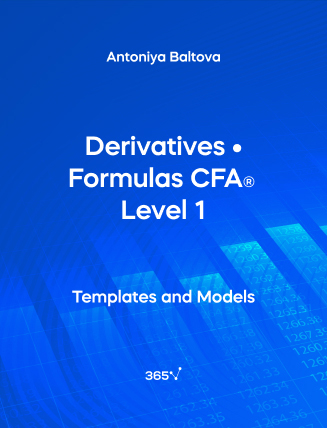## Derivatives • Formulas CFA® Level 1###### Antoniya Baltova

Need an all-in-one list with the Derivatives formulas included in the CFA Level 1 Exam? We have compiled them for you here. The relevant formulas have been organized and presented by chapter. In this section, we will cover the following topics — Replication, Forwards, Swaps, Options, and the Binomial Model.

## 1. Replication

Long risk-free asset (lending)

Long~risk–free~asset~(lending) = Long~asset + Short~derivative

Long derivative

Long~derivative = Long~asset + Short~risk–free~asset~(borrowing)

Short asset

Short~asset = Short~derivative + Short~risk–free~asset~(borrowing)

## 2. Forwards

FRA settlement to the long

FRA~settlement~to~the~long= \frac {Notional~principal \times (Floating~rate - Forward~rate) Big( \frac {Days}{360} Big)}{1 + Floating Big( \frac {Days}{360} Big)}

Days = Number of days in floating rate term
Floating = Floating rate
Forward = Forward rate

Forward rates

F_0(T) = S_0 (1 + r)^T

F_0(T) = Price of the Forward
S_0 = Spot price of the underlying asset
r = Risk-free interest rate
T = Time of the contract

Value

V_T(T) = S_T - F_0(T)

V_T(T) = Value of forward at time T
S_T = Spot price of the underlying at time T
F_0(T) = Price of the Forward

Net Cost of Carry

Net~Cost~of~Carry = ϒ - θ

ϒ = Benefits
θ = Costs

Forward Price with Net Cost of Carry

F_0(T) = (S_0 - ϒ + θ)(1 + r)^T

F_0(T) = Price of the Forward
S_0 = Spot price of the underlying asset
r = Risk-free interest rate
T = Time of the contract
ϒ = Benefits
θ = Costs

Value at any point during the contract (time t)

V_t(T) = S_t - F_0(T)(1 + r)^{-(T - t)}

V_t(T) = Value at time t
S_t = Spot price of the underlying asset at time t

## Call Options

In-the-money: S_T > X
At-the-money: S_T = X
Out-of-the-money: S_T < X

C_T = Call option’s value at expiration (T)
S_T = Stock price at expiration (T)
X = Option’s exercise/strike price
Pi = Profit

## Put Options

In-the-money: S_T < X
At-the-money: S_T = X
Out-of-the-money: S_T > X

P_T = Put option’s value at expiration (T)
S_T = Stock price at expiration (T)
X = Option’s exercise/strike price
Pi = Profit

Put-Call Parity

S_0 + p_0 = c_0 + \frac {X}{(1 + r)^T}

S_0 = Spot price of the underlying asset at time 0
p_0 = Value of put option at time 0
c_0 = Value of call option at time 0
T = Option’s duration
X = Exercise price of the option
r = Risk-free interest rate

Put-Call Forward Parity

\frac {F_0(T)}{(1 + r)^T} + p_0 = c_0 + \frac {X}{(1 + r)^T}

F_0(T) = Forward price
p_0 = Value of put option at time 0
c_0 = Value of call option at time 0
T = Option’s duration
X = Exercise price of the option
r = Risk-free interest rate

## 4. Binomial Model

Up-factor

u = \frac {S_1^+}{S_0}

u = Up-factor
S_1^+ = Upward value of the underlying asset after first period
S_0 = Value of underlying at time 0

Down-factor

d = \frac {S_1^-}{S_0}

d = Down-factor
S_1^- = Downward value of the underlying asset after first period
S_0 = Value of underlying at time 0

Value of option on upward movement

c_1^+ = Max (0, S_1^+ - X) = S_1^+ - X

X = Exercise price of the option
c_1^+ = Option’s value after upward movement

Value of option on downward movement

c_1^- = Max (0, S_1^- - X) = 0

X = Exercise price of the option
c_1^- = Option’s value after downward movement

Synthetic probabilities

c_0 = \frac {πc_1^+ + (1 - π)c_1^-}{1 + r}

c_0 = Value of call option
1 - π = Synthetic probability of downward move

## 5. Swaps

Plain vanilla interest rate swap

Fixed–rate~payment~(t) = (Swap~FR - LIBOR) \times \frac {T}{360} \times NP

FR = Fixed rate
T = Number of days in the settlement period
NP = Notional principal

Follow the links to find more formulas on Quantitative Methods, Economics, Corporate Finance, Alternative Investments, Financial Reporting and Analysis, Portfolio Management, Equity Investments, and Fixed-Income Investments, included in the CFA® Level 1 Exam.Top